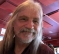## Spectral distortion measure - Itakura-saito distanceStarted by 4 years ago2 replieslatest reply 4 years ago520 views

Hi,

Can someone tell me if Itakura-saito distance calculated in this way gives the value in dB?

If no, then any opinion or suggestion?

Thanks,

Pramod

[ - ]Hello Pramod,

dB computations or calculations are in addition or subtraction.

In fact, that is one of the two reasons going to logarithm in first place.

The equation mentioned is an integral with typical metrics (scalar not dB).

The integral is over d(omega) and then divided by 2pi, so the result is frequency, Hz.

Shahram Shafie

[ - ]